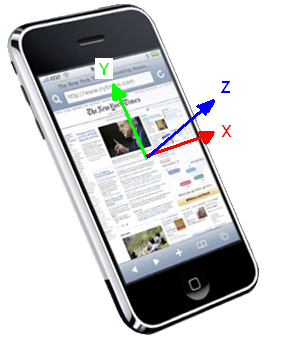# How to convert Input.GetAxis to Accelerometer control?

Trying to convert this script for mobile use. How can I change the input.GetAxis statements to recognize accelerometer control rather than keyboard, I have tried a couple ways and cant get it, can someone get me over this hump? THANKS!!

``````// A very simplistic car driving on the x-z plane.

var speed : float = 10.0;
var rotationSpeed : float = 100.0;

function Update () {
// Get the horizontal and vertical axis.
// By default they are mapped to the arrow keys.
// The value is in the range -1 to 1
var translation : float = Input.GetAxis ("Vertical") * speed;
var rotation : float = Input.GetAxis ("Horizontal") * rotationSpeed;

// Make it move 10 meters per second instead of 10 meters per frame...
translation *= Time.deltaTime;
rotation *= Time.deltaTime;

// Move translation along the object's z-axis
transform.Translate (0, 0, translation);
// Rotate around our y-axis
transform.Rotate (0, rotation, 0);
}
``````

You’re not so far! According to the Input script reference, in mobile devices Unity assumes Y parallel to the long side, X parallel the short side and Z pointing to the user, like this:You can do the following: when the game starts, save the current Input.acceleration as a zero reference; during the game, subtract Input.acceleration from this reference and use the resulting X and Y to feed the Horizontal and Vertical axes (or vice versa, if the button is at the right side during the game):

``````var zeroAc: Vector3;
var curAc: Vector3;
var sensH: float = 10;
var sensV: float = 10;
var smooth: float = 0.5;
var GetAxisH: float = 0;
var GetAxisV: float = 0;

function ResetAxes(){
zeroAc = Input.acceleration;
curAc = Vector3.zero;
}

function Start(){
ResetAxes();
}

function Update(){
curAc = Vector3.Lerp(curAc, Input.acceleration-zeroAc, Time.deltaTime/smooth);
GetAxisV = Mathf.Clamp(curAc.y * sensV, -1, 1);
GetAxisH = Mathf.Clamp(curAc.x * sensH, -1, 1);
// now use GetAxisV and GetAxisH instead of Input.GetAxis vertical and horizontal
// If the horizontal and vertical directions are swapped, swap curAc.y and curAc.x
// in the above equations. If some axis is going in the wrong direction, invert the
// signal (use -curAc.x or -curAc.y)
}
``````